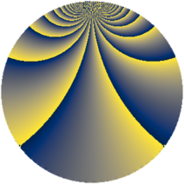# Properties

 Label 1380.2.hLevel $1380$ Weight $2$ Character orbit 1380.h Rep. character $\chi_{1380}(1151,\cdot)$ Character field $\Q$ Dimension $176$ Sturm bound $576$

# Related objects

## Defining parameters

 Level: $$N$$ $$=$$ $$1380 = 2^{2} \cdot 3 \cdot 5 \cdot 23$$ Weight: $$k$$ $$=$$ $$2$$ Character orbit: $$[\chi]$$ $$=$$ 1380.h (of order $$2$$ and degree $$1$$) Character conductor: $$\operatorname{cond}(\chi)$$ $$=$$ $$12$$ Character field: $$\Q$$ Sturm bound: $$576$$

## Dimensions

The following table gives the dimensions of various subspaces of $$M_{2}(1380, [\chi])$$.

Total New Old
Modular forms 296 176 120
Cusp forms 280 176 104
Eisenstein series 16 0 16

## Trace form

 $$176q + 6q^{6} + 8q^{9} + O(q^{10})$$ $$176q + 6q^{6} + 8q^{9} - 14q^{12} + 16q^{13} - 26q^{18} - 24q^{22} + 4q^{24} - 176q^{25} - 24q^{28} - 16q^{30} + 32q^{33} + 24q^{34} + 10q^{36} - 16q^{37} + 64q^{42} + 38q^{48} - 224q^{49} + 60q^{52} + 20q^{54} - 48q^{57} + 36q^{58} + 28q^{60} - 16q^{61} + 12q^{64} - 12q^{66} + 24q^{70} - 44q^{72} + 16q^{76} - 6q^{78} - 40q^{81} + 4q^{82} - 88q^{84} - 40q^{88} - 36q^{90} - 48q^{93} - 36q^{94} + 30q^{96} + 96q^{97} + O(q^{100})$$

## Decomposition of $$S_{2}^{\mathrm{new}}(1380, [\chi])$$ into newform subspaces

The newforms in this space have not yet been added to the LMFDB.

## Decomposition of $$S_{2}^{\mathrm{old}}(1380, [\chi])$$ into lower level spaces

$$S_{2}^{\mathrm{old}}(1380, [\chi]) \cong$$ $$S_{2}^{\mathrm{new}}(60, [\chi])$$$$^{\oplus 2}$$$$\oplus$$$$S_{2}^{\mathrm{new}}(276, [\chi])$$$$^{\oplus 2}$$